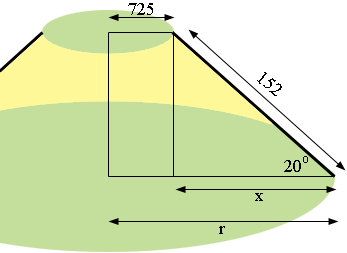SEARCH HOMEMath Central Quandaries & QueriesQuestion from Lucian: I need to calculate the bottom inside diameter of a truncated cone. The top insdie diameter is 1450mm. The material is 6mm thick The cone angle is 20 degrees The slant length is 152mm I would like a formula so that I can build a spread sheetLucian,

Here is my diagram (not to scale) the dimensions are in millimeters., I hope it illustrates your situation.From the diagram x/152 = cos(20o) and hence

x = 152 × cos(20o) = 152 × 0.9397 = 143 mm.

Thus the inside radius of the base is

r = 725 + 143 = 868 mm.

I hope this helps,
PennyMath Central is supported by the University of Regina and The Pacific Institute for the Mathematical Sciences.